# 霍格沃兹测试学院 上海线下一期_python (作业)_20180623

1.使用python写一个获取当前日期的函数，获取到的日期按照(年-月-日)排列； (提示：导入time或datetime库)

2.使用python写一个获取当前系统时间的函数，获取到的时间以(时:分：秒)排列； (提示：导入time或datetime库)

3.使用python写一个函数，具体逻辑如下：

(提示：导入os库)

``import timet = time.localtime()year = t.tm_yearmonth = t.tm_monday = t.tm_mdayhour = t.tm_hourmin = t.tm_minsec = t.tm_secprint("当前日期为：%s-%s-%s" % (year, month, day))print("当前时间为：%s:%s:%s" % (hour, min, sec))``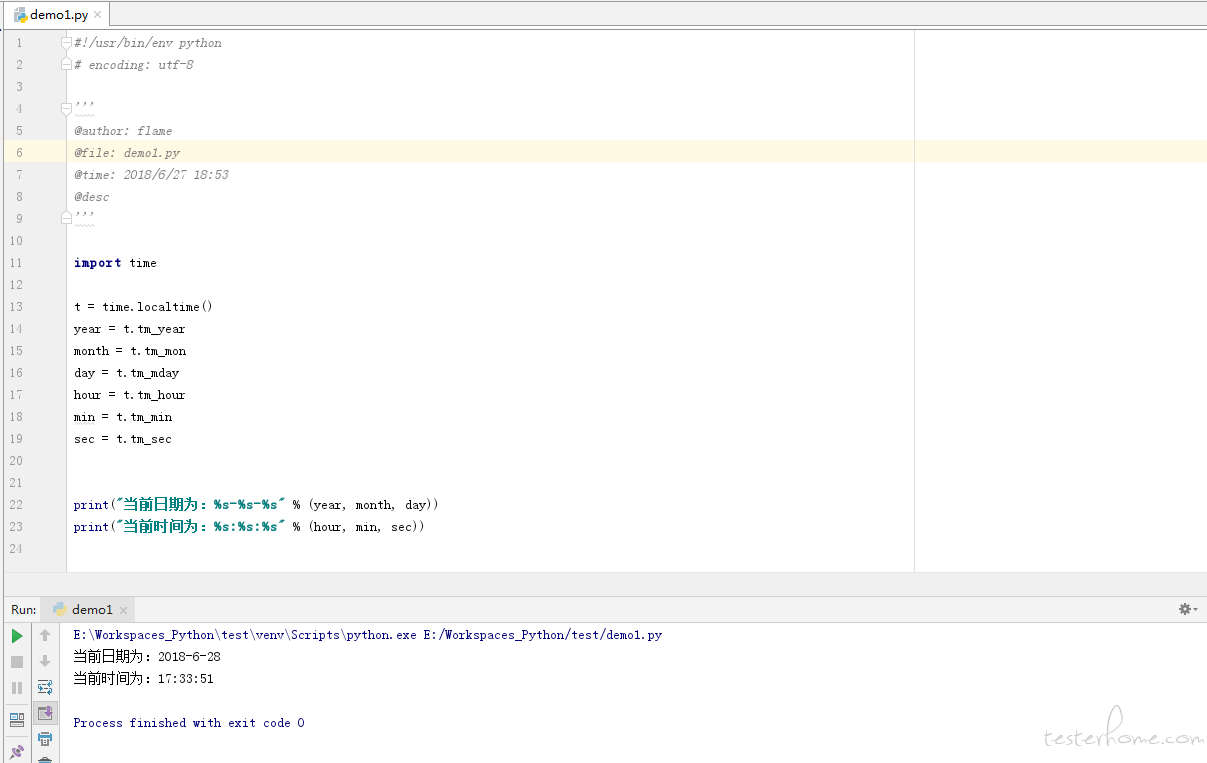``import osfile = input("请输入一个有效的目录地址：")try:    if os.path.exists(file):        print("%s 已经存在该系统中" %os.path.abspath(file))    else:        print("该目录不存在，正在创建中。。。")        os.mkdir(file)        print("现在 %s 已经存在该系统中" %os.path.abspath(file))except Exception as e:    print(e)``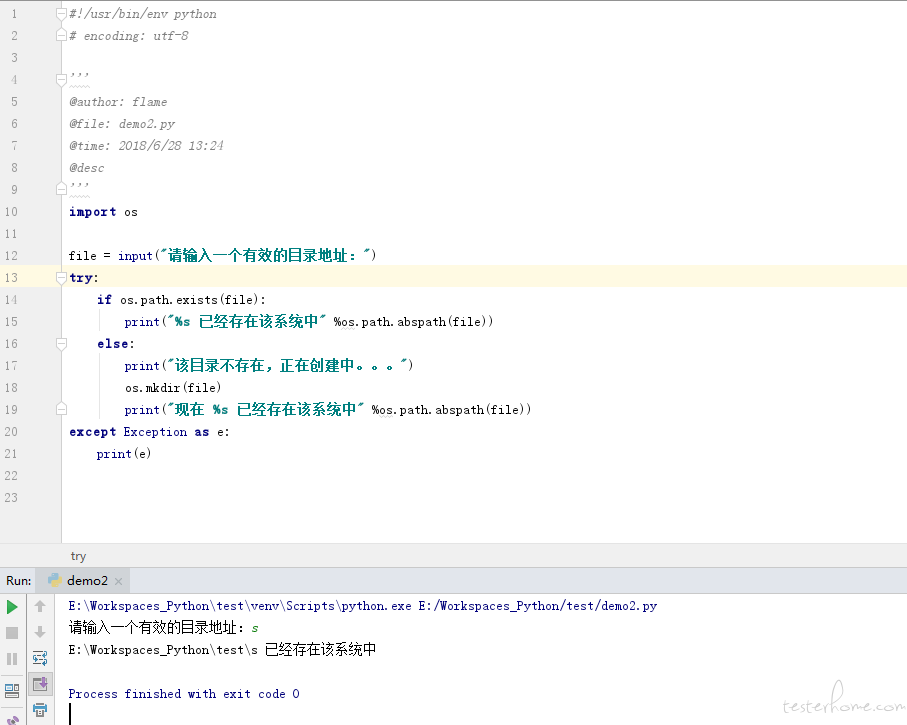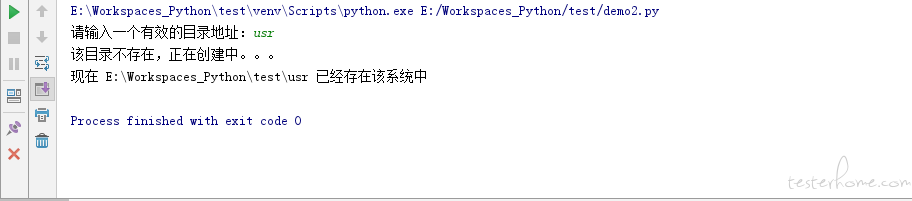``#! /usr/bin/python# --*-- coding:utf-8 --*--from datetime import datetimenow = datetime.now()print(now.strftime('%Y-%m-%d'))``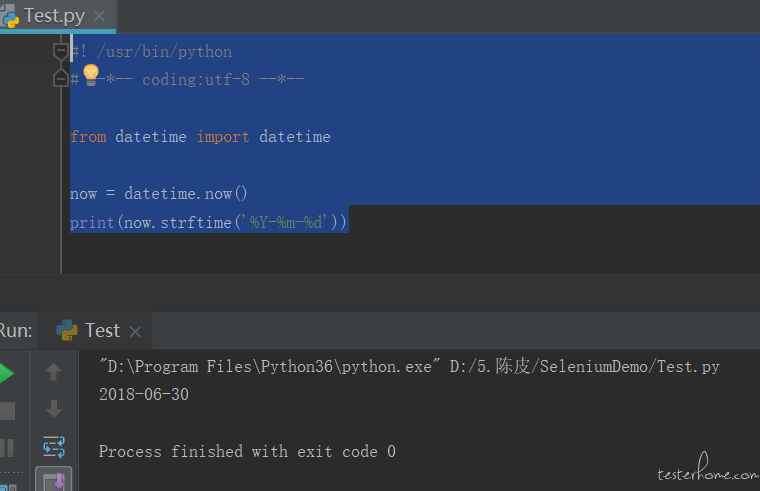``#! /usr/bin/python# --*-- coding:utf-8 --*--from datetime import datetimenow = datetime.now()print(now.strftime('%H:%M:%S'))``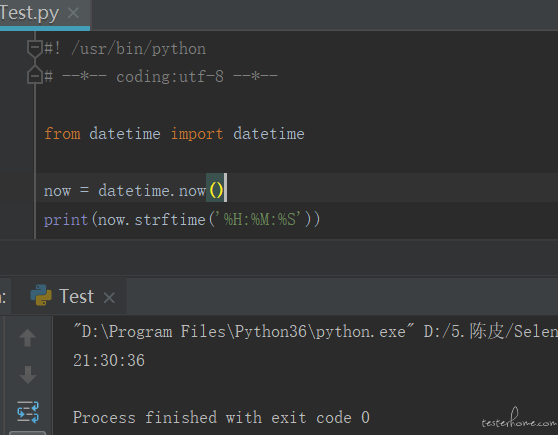``#! /usr/bin/python# --*-- coding:utf-8 --*--import osdef dir(path):    if os.path.isdir(path):        print('direct exist')        return path    else:        os.mkdir(path)        print('create direct')        return pathdir('C:\\AMD\\WU-CCC2')dir('C:\\AMD\WU-CCC2\\test')``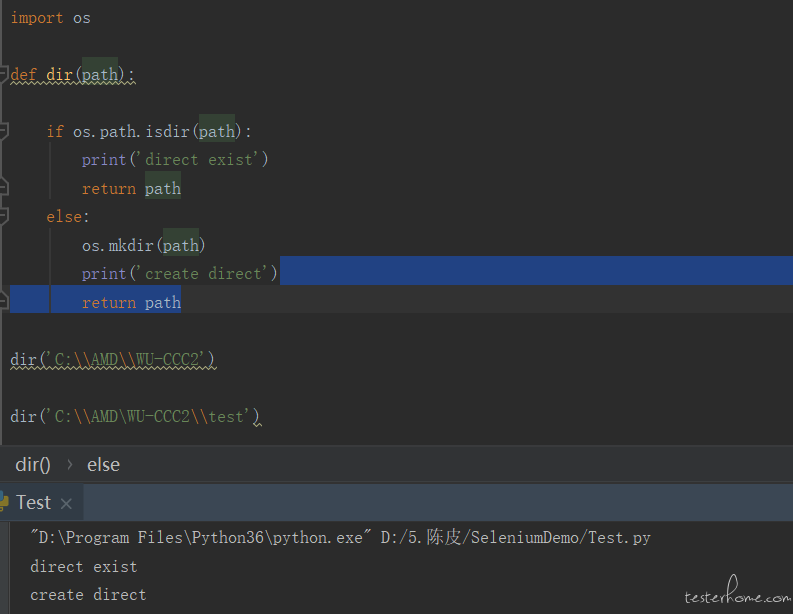``dir(r"C:\Users\MyPC\PycharmProjects\testerhome\\")``

``import osdef jude_dir(path):#dir是内置的方法名    "处理目录，如果有返回目录名称，如果没有创建文件夹"    #路径a 条件表达式    if not os.path.exists(path):#判断如果不存在        os.mkdir(path) #创建        print('create direct')    #路径b    else:        print('direct exist')    #无论走到哪里都会走到下面    return path ``
``import timeclass Base(object):    def local_day(self):        "年:月:日"        return time.strftime("%Y-%m-%d", time.localtime())    def local_time(self):        "小时:分钟:秒"        return time.strftime("%H:%M:%S", time.localtime())b = Base()print("当前日期单位", b.local_day())print("当前时间单位", b.local_time())``

``def Date_Time():    import time    date = time.strftime("%Y-%m-%d", time.localtime())    time = time.strftime("%H:%M:%S", time.localtime())    print("当前的日期是：", date)    print("当前的时间是：", time)Date_Time()``

``import osfilename = r'D:\assignments\a'if os.path.exists(filename):    catalog = '文件目录为"%s"'else:    os.makedirs(filename)    catalog = '新建文件目录为"%s"'print(catalog % filename)``